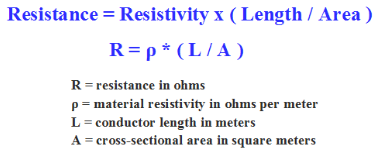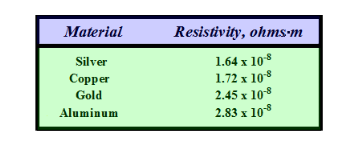# The Significance Of Conductor Resistance And How To Calculate It

In this installment of the "Practicing Technician" series we will review the calculation required to determine the resistance of a given conductor. This often overlooked parameter can be important when trying to determine the appropriate wire diameter for a given application. It is also important to consider conductor resistance when examining application efficiency. Lower resistance means lower power dissipation by the conductor. Optimizing these two aspects of conductor resistance for your specific application can lead to significant reductions to implementation and operating costs. It is important to know the resistance offered by a given conductor as well as have an understanding of the extent to which that resistance impacts on the application and its operation. For this reason, we will review some relevant aspects of conductor resistance and briefly describe and discuss them.

## What factors determine the resistance of a given conductor?

There are three factors that determine the amount of resistance a given conductor will have. They are illustrated here, in the relationship used to calculate conductor resistance.We begin by acknowledging the obvious fact that the length of a conductor will contribute to its overall resistance. The longer the length of a given conductor, the more resistance that conductor will have. This can be clearly seen in the relationship provided above.

The material resistivity of the conductor plays a significant role in the overall resistance. This is because different materials for example gold or copper, offer different amounts of resistance to continuous current flow. Conductor materials are generally selected on a cost-benefit and suitability basis. The material resistivity of some of the most common conductors used today is listed below.The last significant factor that determines conductor resistance is the cross-sectional area of the given conductor. It is important to note the inverse relationship between the cross-sectional area of a conductor, and the conductor’s resistance. As evidenced by the provided example, the smaller the cross-sectional area of the conductor, the larger the resistance value of the conductor becomes. This means that although it may be cheaper to use smaller conductor sizes, there is a trade off with resistance.

## In what ways can conductor resistance be significant?

This relationship provides a means of determining conductor resistance which can be used to examine power losses that contribute to the overall efficiency of an application. This relationship can also be rearranged to solve for Area. This calculated value for cross-sectional area can then be used to provide the required wire diameter to meet specific application needs. In the video animation provided in the link below, we examine the steps required to determine the minimum wire diameter that can be used when given specific criteria pertaining to conductor length and acceptable conductor resistance. As previously stated, these two considerations have impact on implementation and operating costs and are worth examining.

If you liked this post, check out our previous articles in The Practicing Technician’s Series;

We hope this has been helpful to you as a practicing or student technician. We are looking for your feedback or other ideas for the Practicing Technician’s Series. Please let us know your ideas about what you would like us to write about by sending us your thoughts and questions to support@gbctechtraining.com.